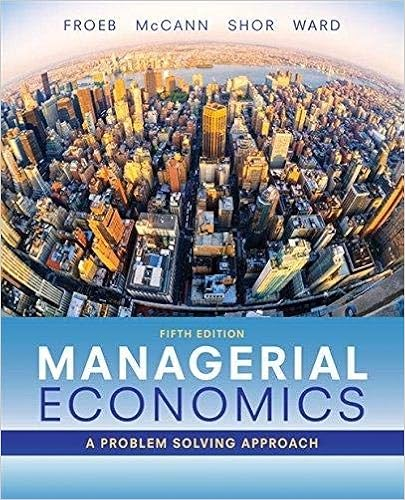# Refer to figure 15 4 what is the profit

• Test Prep
• 19
• 92% (36) 33 out of 36 people found this document helpful

This preview shows page 11 - 13 out of 19 pages.

##### We have textbook solutions for you!
The document you are viewing contains questions related to this textbook.The document you are viewing contains questions related to this textbook.
Chapter 10 / Exercise 10
Managerial Economics
Froeb/McCannExpert Verified
57) Refer to Figure 15 - 4. What is the profit - maximizing/loss - minimizing output level? 57) A) 600 units B) 800 units C) 940 units D) 1,160 units
58) Refer to Figure 15 - 4. What is the price charged for the profit - maximizing output level? 58)
59) Refer to Figure 15 - 4. What is the amount of the monopoly's total revenue? 59)
60) Refer to Figure 15 - 4. What is the amount of the monopoly's total cost of production? 60)
11
##### We have textbook solutions for you!
The document you are viewing contains questions related to this textbook.The document you are viewing contains questions related to this textbook.
Chapter 10 / Exercise 10
Managerial Economics
Froeb/McCannExpert Verified
61) Refer to Figure 15 - 4. What is the amount of the monopoly's profit? 61) A) \$2,700 B) \$4,200 C) \$10,400 D) \$12,600
62) Refer to Figure 15 - 4. What is likely to happen to this monopoly in the long run? 62)
Table 14 - 2 Table 14 - 2 shows the payoff matrix for Wal - Mart and Target from every combination of pricing strategies for the popular PlayStation 3. At the start of the game each firm charges a low price and each earns a profit of \$7,000. 63) Refer to Table 14 - 2. Is the current strategy in which each firm charges the low price and earns a profit of \$7,000 a Nash equilibrium? If not, why and what is the Nash equilibrium? 63)
64) Refer to Table 14 - 2. For each firm, is there a better outcome than the current situation in which each firm charges the low price and earns a profit of \$7,000? 64)
•••